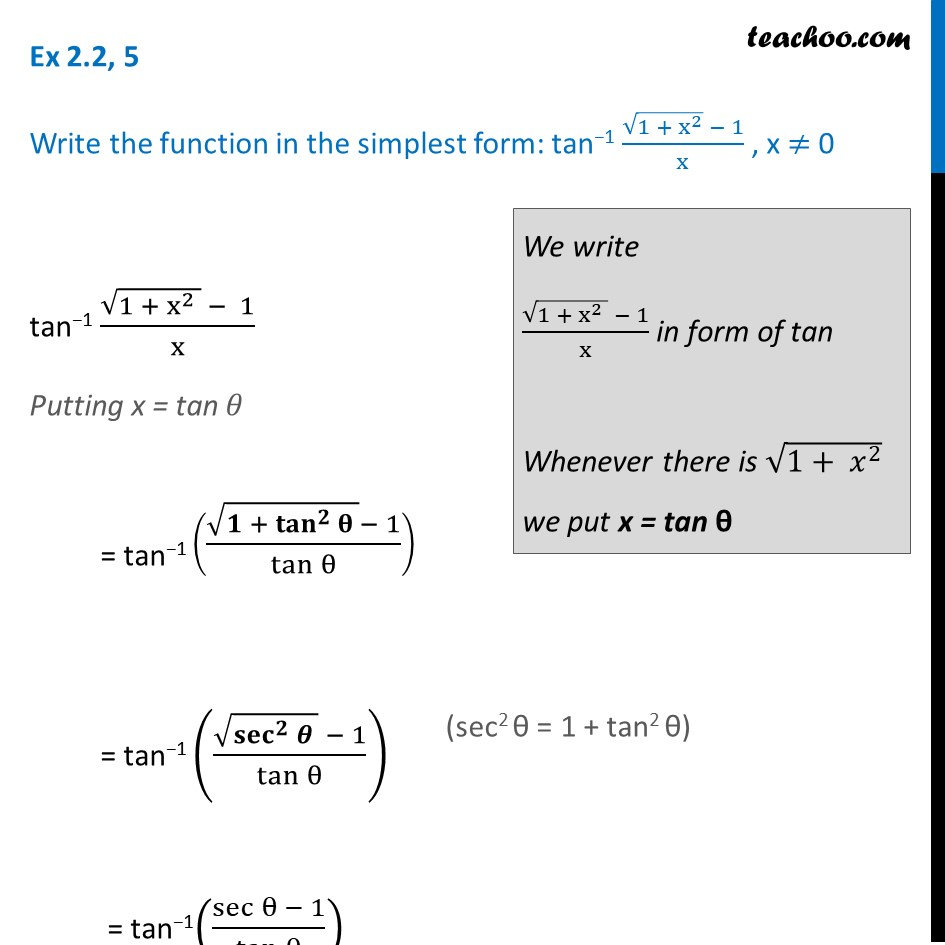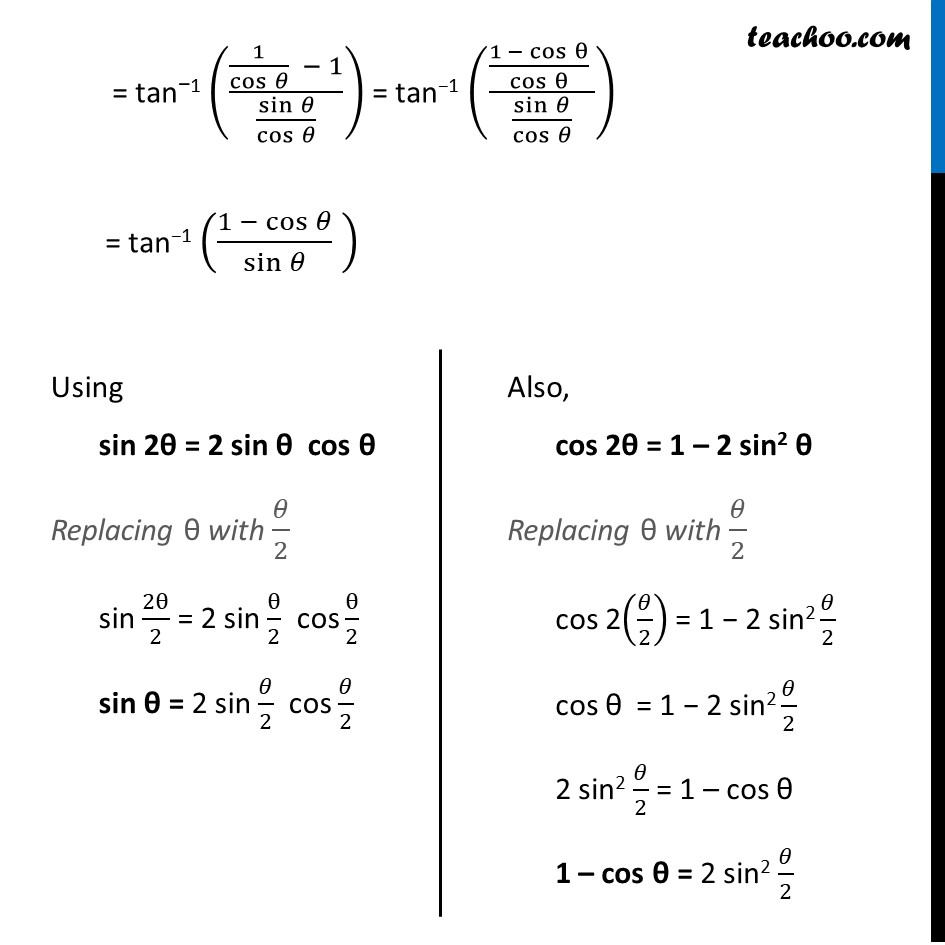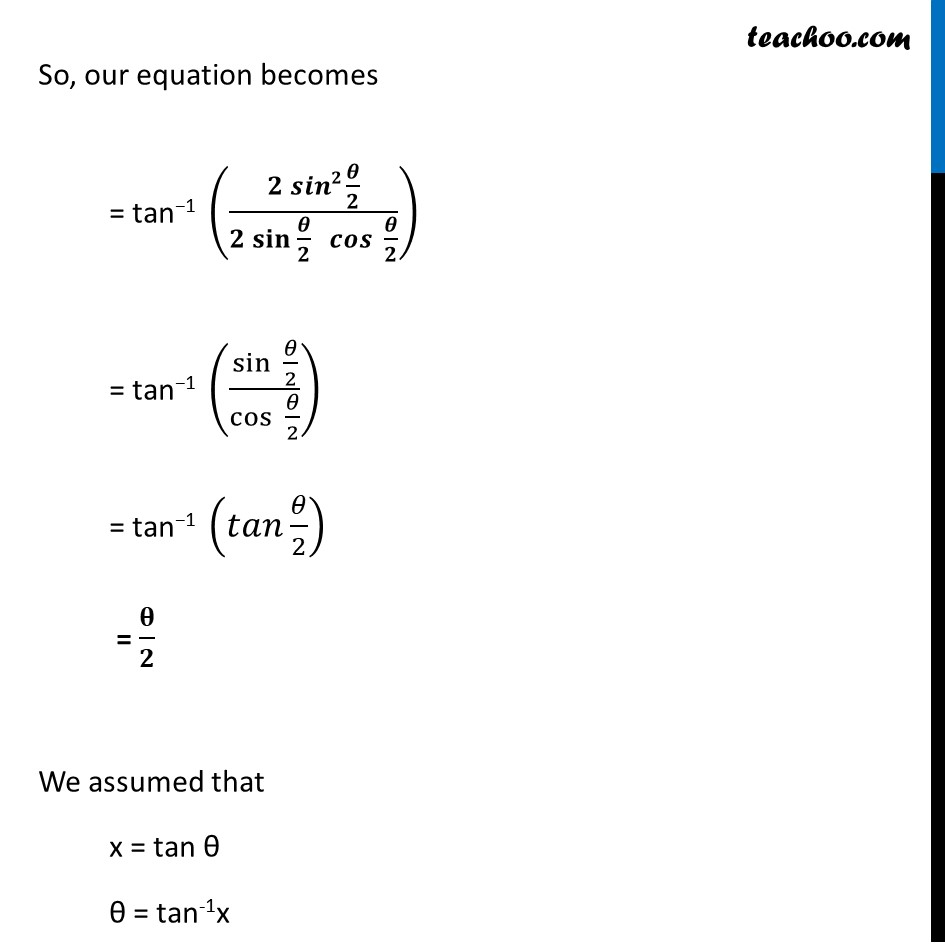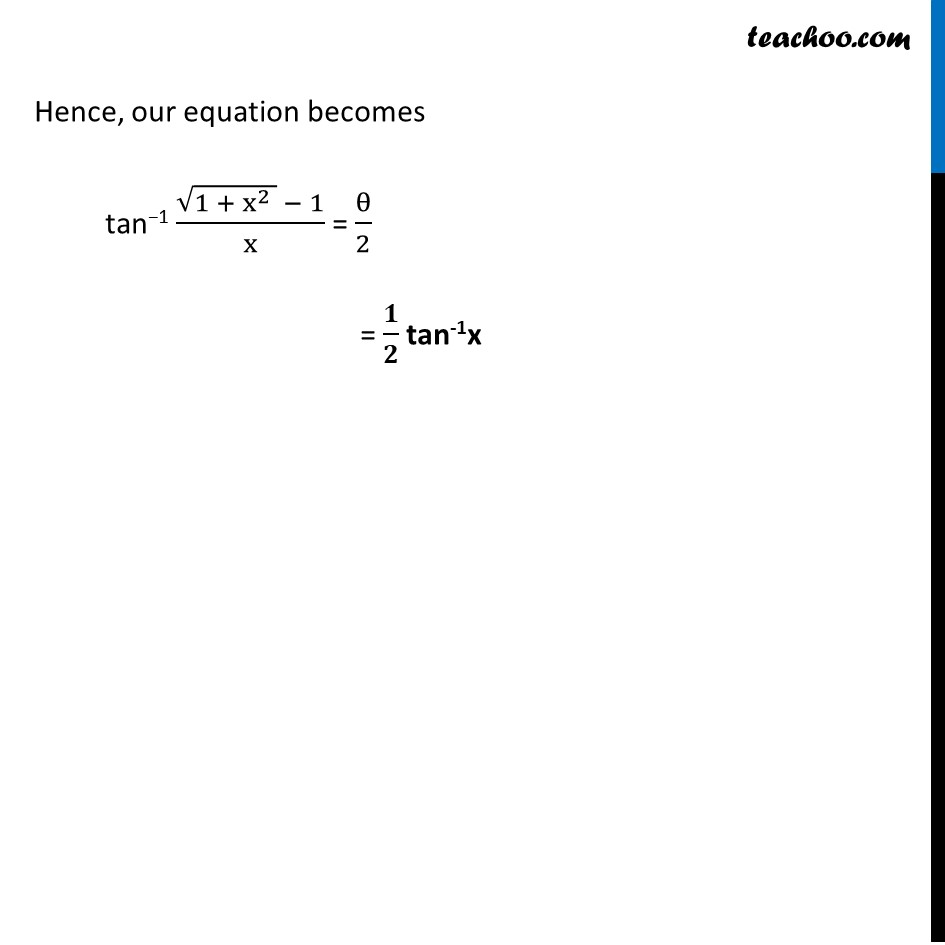Ex 2.2

Chapter 2 Class 12 Inverse Trigonometric Functions (Term 1)
Serial order wise### Transcript

Ex 2.2, 5 Write the function in the simplest form: tan−1 (√(1 + x^2 ) − 1)/x , x ≠ 0 tan−1 (√(1 + x^2 ) − 1)/x Putting x = tan 𝜃 = tan−1 ((√(𝟏 + 〖𝐭𝐚𝐧〗^𝟐 𝛉 )− 1)/(tan θ)) = tan−1 ((√(〖𝐬𝐞𝐜〗^𝟐 𝜽 ) − 1)/(tan θ)) = tan−1((sec⁡θ − 1)/(tan θ)) We write (√(1 + x^2 ) − 1)/x in form of tan Whenever there is √(1+ 𝑥^2 ) we put x = tan θ (sec2 θ = 1 + tan2 θ) = tan−1 ((1/cos⁡𝜃 − 1)/(sin⁡𝜃/cos⁡𝜃 )) = tan−1 (((1 − cos⁡θ)/cos⁡θ )/(sin⁡𝜃/cos⁡𝜃 )) = tan−1 ((1 −〖 cos〗⁡𝜃)/sin⁡𝜃 ) Using sin 2θ = 2 sin θ cos θ Replacing θ with 𝜃/2 sin 2θ/2 = 2 sin θ/2 cos θ/2 sin θ = 2 sin 𝜃/2 cos 𝜃/2 Also, cos 2θ = 1 – 2 sin2 θ Replacing θ with 𝜃/2 cos 2(𝜃/2) = 1 − 2 sin2 𝜃/2 cos θ = 1 − 2 sin2 𝜃/2 2 sin2 𝜃/2 = 1 – cos θ 1 – cos θ = 2 sin2 𝜃/2 So, our equation becomes = tan−1 ((𝟐 𝒔𝒊𝒏𝟐 𝜽/𝟐)/(𝟐 〖𝐬𝐢𝐧 〗⁡〖𝜽/𝟐〗 𝒄𝒐𝒔⁡〖 𝜽/𝟐〗 )) = tan−1 (〖sin 〗⁡〖𝜃/2〗/cos⁡〖 𝜃/2〗 ) = tan−1 (𝑡𝑎𝑛 𝜃/2) = 𝛉/𝟐 We assumed that x = tan θ θ = tan-1x Hence, our equation becomes tan−1 (√(1 + x^2 ) − 1)/x = θ/2 = 𝟏/𝟐 tan-1x